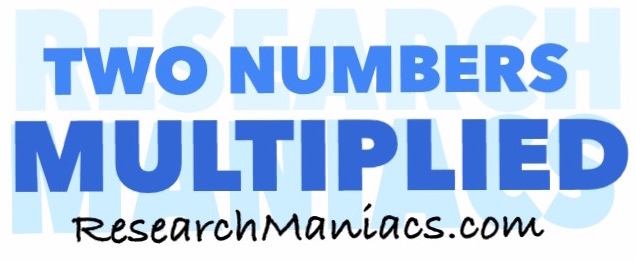What two numbers multiply to 4?What 2 numbers do you multiply to get 4? In other words, what number can you multiply with another number to get 4?

The formula to solve this would be:

X x Y = 4

There are at least two combinations of two numbers that you can multiply together to get 4.

For your convenience, we have made a list of all the combinations of two numbers multiplied by each other that will make 4:

1 x 4 = 4
2 x 2 = 4
4 x 1 = 4

Two Numbers Multiplied
Enter another number below to see which combinations of two numbers multiplied will equal that number.

What two numbers multiply to 5
Go here for the next number on our list.